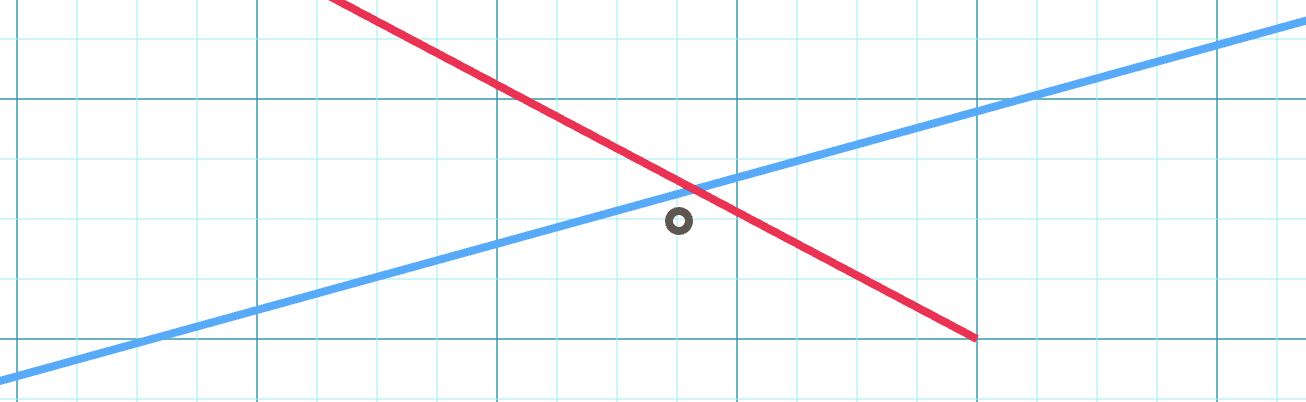# Why does the intersection of A and B not intersect A or B?

The intersection of two lines, A and B, is a point P. In math class, you learn that P falls on both A and B. It’s just math!

So, why are there easily constructable cases in PostGIS where the intersection of A and B does not intersect either input line?

``````WITH parts AS (
SELECT
'LINESTRING(0 0, 10 11)'::geometry AS a,
'LINESTRING(11 0, 0 19)'::geometry AS b
)
SELECT
ST_AsText(ST_Intersection(a, b)) AS intersection,
ST_Intersects(ST_Intersection(a, b), a) AS intersects_a,
ST_Intersects(ST_Intersection(a, b), b) AS intersects_b
FROM parts;
``````
``````intersection | POINT(6.720257234726688 7.392282958199357)
intersects_a | f
intersects_b | f
``````

Coordinates in PostGIS are represented as pairs of “floating point” numbers, which are capable of representing a very large numner of values. But not an infinite number of values.

The rule that “where A intersects B at P, P will be on both A and B” is a mathematical rule for the “real numbers” of which there are an infinite number. The finite quantity of numbers that a computer’s floating point can represent end up looking like a piece of graph paper.If you draw a straight line on a piece of graph paper, mostly the line won’t hit many graph crossing points. The same is true of lines in the world of the “floating point grid”. The result is that the calculated intersection of two lines, frequently does not land on either of the lines, but instead lands on a (very very close) nearby point that is representable as a floating point number.